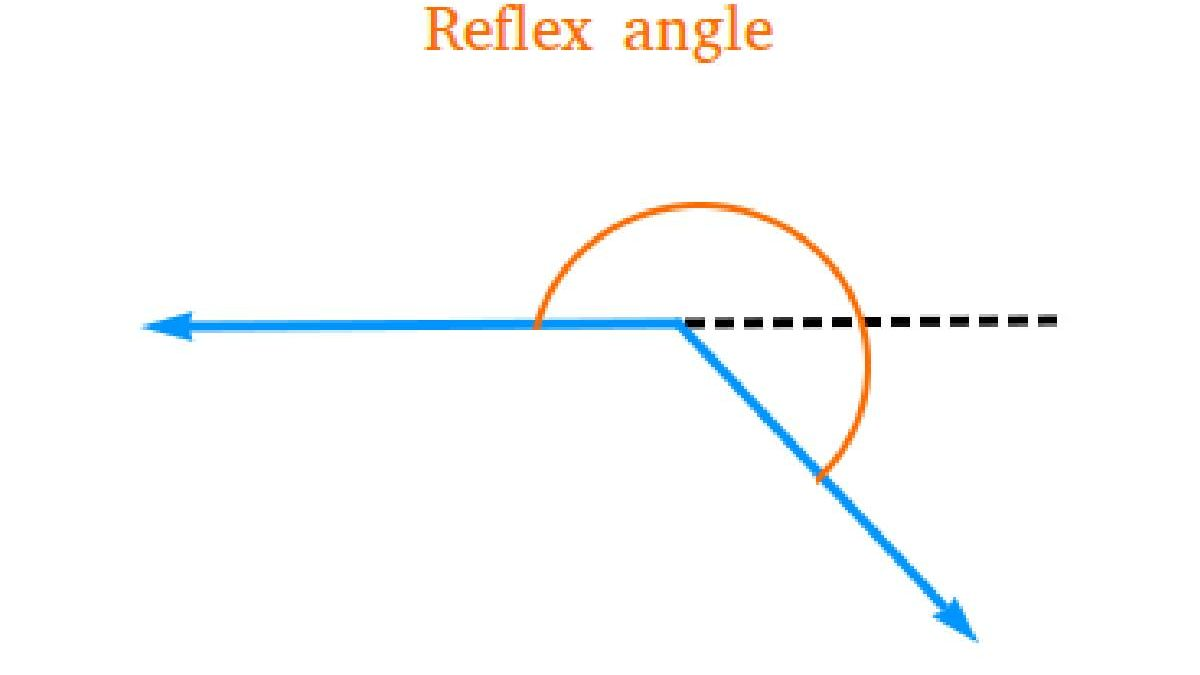### Trending Articles# Reflex angle and their components

What is a reflex angle? It is an angle that is greater than 180 degrees but it would be less than 360 degrees. It is also known to have a corresponding angle which lies on the other side of it. There is a need to educate ourselves about reflex angle before we go ahead.

## More about a reflex angle

As already mentioned a reflex angle is one that is between 180 degrees to 360 degrees. An illustration in the form of a figure will provide you with a comprehensive idea on what a angle is all about.

A reflex angle would always be greater than a straight angle, when it is a half circle is at 180 degrees. At a full circle it would be 360 degrees. A point to consider is that a reflex angle would always be having an acute angle, a right angle or an obtuse angle on the opposite side of it.

The angle and the corresponding angle would be adding up to 360 degrees. It is therefore easy to calculate the angles of a reflex angle. An example is if an acute angle measure 56 degrees, then the corresponding angle would turn out to be 360- 56= 304 degrees. On the other hand if an obtuse angle would measure 87 degree then the corresponding angle would be 360- 87= 273 degrees. When a couple of lines intersect at a point, the measure of the opening between both angles is known as an angle

## The ways by which you may measure a reflex angle

It is obvious that with the aid of a proctor we may be able to measure a reflex angle. By following the below mentioned steps you may be able to measure a reflex angle

• With the help of a protractor you would need to measure the internal angle. Here the measure of an internal angle is 110 degrees
• Then you need to subtract this angle from 360 degrees that is going to provide you with the value of the corresponding angle. When you deduct 360 degrees from 110 degrees it would provide you with a value of 250 degrees
• Hence the measurement of a reflex angle is 250 degrees

## Reflex angle in our real life context

It is a common occurrence to witness angles all around us in our daily lives. Hence it would be vital to have an understanding about reflex angles in our day to day activities.

• Clock- if we are observing both hands of a clock at 7 a particular side of the clock develops an obtuse angle. It is a side that would be falling on one side of the numbers 7, 8, 9, 10, 11 or 12. The other side that has the numbers 1 to 6 would be forming the reflex angle
• In a circular pizza, if we would be cutting a small slice and remove a portion of it, then the pizza that is remaining forms a reflex angle
• A rectangular tab would be forming a right angle of 90 degrees that is the top along with the leg. An outer angle may be performed with the same top
• A table is an example of a reflex angle. It forms a right angle with a leg and it’s top. An outer angle that is formed with the same top and leg that is at a reflex angle of 270 degrees

The best way to gain insights about this angle is via website like Cuemath. They provide a comprehensive analysis on how to cope up with the subject matter easily.